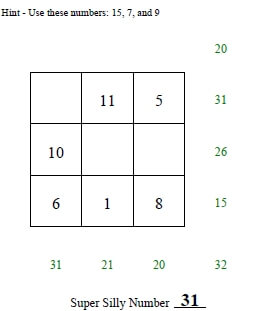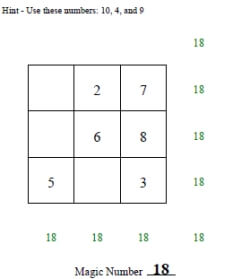Worksheets and No Prep Teaching Resources
Math Worksheets

# Super Silly Squares

Super silly squares are anything but silly as they stretch students' minds and mathematical skills to solve problems and complete the square. Students will be delighted to expand their math operations skills with an activity that seems more like a game than math practice. A wide range of these creative squares will keep students at all levels happily solving math problems while having a lot of fun.3 by 3 Super Silly Squares

Numbers 1 to 9 - No Missing Sums
Numbers 1 to 9 - With Missing Sums
Mix of Positive Numbers - With Missing Sums
Mix of Negative Numbers - With Missing Sums

3 by 3 Magic Squares and Super Silly Squares Mix

3 by 3 Magic Squares and Super Silly Squares - Numbers 1 to 9
3 by 3 Magic Squares and Super Silly Squares - Mix of Different Positive NumbersPositive numbers: 2 blanks (first answer given; hint for numbers to use given) Positive numbers: 2-3 blanks (first answer given; hint for numbers to use given) Positive numbers: 2-3 blanks (first answer given; hint for numbers to use given; 3 pages for each key) Positive numbers: 3-4 blanks (first answer given; hint for numbers to use given)

3 by 3 Multiplication Super Silly Squares

Numbers 1 to 9 - No Missing Sums
Numbers 1 to 9 - With Missing Sums
Mix of Positive Numbers - With Missing Sums

Subtraction Squares

Multiplication Squares

Mixed Math Square Puzzles

Magic Square Puzzles

Have a suggestion or would like to leave feedback?